# Altova MobileTogether Designer

 Action Groups with Action-Group Parameters Scroll Home Prev Top Next More

Action Groups can use two types of parameters:

Simple parameters, which are described in the section Action Groups with Parameters. They are indicated by the dollar symbol \$ in front of their names.

Action-Group parameters, which take Action Groups as their values. They are indicated by the percent symbol % in front of their names.

In the screenshot below, \$length and \$width are simple parameters, while %RectangleProperty is an Action-Group parameter. To create a parameter as an Action-Group parameter, create it as a simple parameter and then check its Action Group check box (see screenshot below).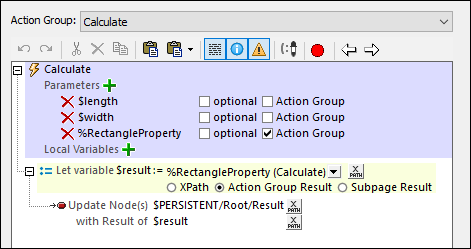## How Action-Group parameters work

An Action-Group parameter is a parameter whose value is an Action Group that declares no parameter. This enables the called Action Group to be treated like a function that performs MobileTogether tasks. Action-Group parameters work as follows:

In an Action Group, declare Action-Group parameters. These parameters can be used in actions of the Action Group. In the screenshot above, for example, the Calculate Action Group declares the RectangleProperty Action Group to be one of its parameters. The RectangleProperty Action Group thus becomes an Action-Group parameter. It is then used inside the Calculate Action Group.

The values of all parameters of an Action Group (including those of any Action-Group parameter) are supplied when the Action Group is called. This happens when the Execute Action Group action is executed on that Action Group. For example, when we call the Calculate Action Group (shown in the screenshot above) via an Execute Action Group action, we pass it the values of the Action Group's three parameters (see screenshot below).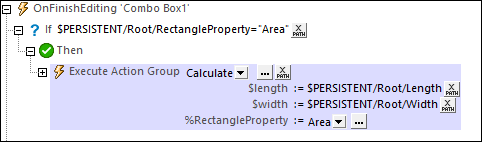## Usage example

Here is a simple example to show how Action-Group parameters can be used. Let us say we want to calculate the area, diagonal length, or perimeter of a rectangle from a user-given length and width (see screenshot below). The user can select which rectangle property to calculate.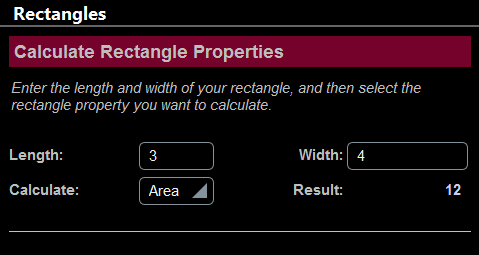For each of the three properties, we can create an Action Group that declares no parameters. In effect, these three Action Groups are used as functions to calculate the respective rectangle property, as shown in the screenshot below.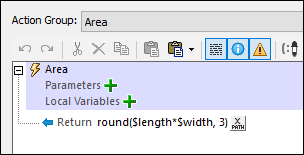We now create a fourth Action Group (named Calculate in our example; see screenshot below) that will use one of the other three Action Groups as required. To select the Action Group e want, we create an Action-Group parameter (named %RectangleProperty in our example). Note that the Calculate Action Group also declares the two simple parameters (\$length and \$width) that are used to calculate the area, diagonals, and perimeter.All that we need to do now is to call the Calculate Action Group via an Execute Action Group action and, in the call, supply the values of the Calculate Action Group's three parameters. The Execute Action Group action is carried out ideally when some event is triggered. For example, in the screenshot below, the action is carried out when a combo box is edited.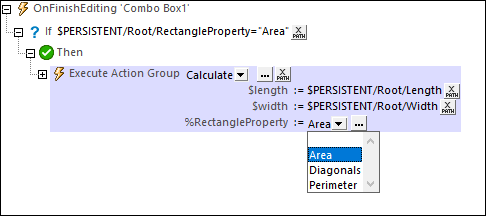What happens when the Execute Action Group action is carried out is this:

1.The Calculate Action Group is called. Values of its \$length and \$width parameters are passed from nodes of the \$PERSISTENT page source (see screenshot above). The values of the RectangleProperty Action-Group parameter must be an Action Group in the project and can therefore be selected via the combo box; any one Action Group (corresponding to a chosen rectangle property) can be selected. In the screenshot of our example (above), we have selected the Area Action Group (in the event that the \$PERSISTENT/Root/RectangleProperty node contains the string "Area").

2.The Calculate Action Group is now executed with the parameter values passed to it. If you look at its screenshot above, you will see that it defines a variable named \$result and gives it a value that is the return value of the Action Group specified by the %RectangleProperty parameter. This variable will therefore contain the value of the selected rectangle property (area, diagonal length, or perimeter).

3.The Calculate Action Group now updates a node in the \$PERSISTENT tree with this result.

### Details of the Action Group definitions

Calculate (see screenshot above): Declares two simple parameters (\$length and \$width) and an Action-Group parameter (%RectangleProperty). The Action-Group parameter %RectangleProperty can take as its value any of the other three Action Groups, all of which are defined without any parameter. In the Calculate Action Group, we define a Let action that is set to Action Group Result. The Let action defines a variable called \$result, which is defined to take as its value the result of executing the selected Action Group.

Area (screenshot below): This Action Group contains a single Return action that contains the product of the two simple parameters \$length and \$width. This Action Group contains no parameter; it can therefore be the value of %RectangleProperty. XPath to calculate area: round((\$width*\$length), 3).Diagonals: This Action Group is similar to the Area Action Group. It contains a single Return action that returns the length of the diagonals of a rectangle (which is the square root of the sum of the squares of the two simple parameters \$length and \$width; XPath: round(math:sqrt(\$width*\$width \$length*\$length), 3)).

Perimeter: This Action Group is similar to the Area and Diagonals Action Groups. It contains a single Return action that returns the perimeter of a rectangle (XPath: round((2*\$width + 2*\$length), 3)).

In the description of the four Action Groups above, note the following:

The Calculate Action Group contains three parameters: two simple parameters and one Action-Group parameter.

The other three Action Groups (Area, Diagonals, Perimeter) declare no parameter.

Each of the three Action Groups Area, Diagonals, Perimeter has a Return action that performs a calculation. The returned value is the Action Group Result.

Each of the three Action Groups Area, Diagonals, Perimeter can be set as the value of the Action-Group parameter %RectangleProperty, and would return their respective Action Group Result when processed.

 Note: The example above is deliberately simple; it aims to show the mechanism behind Action-Group parameters. Action-Group parameters, however, are best used with dynamic content and to execute complex actions.

## Processing of Action-Group parameters

An Action-Group parameter takes an Action Group as its value. This Action Group can be processed as a parameter value in two useful ways:

It can provide a result—the Action Group Result—which can then be used to set the value of a variable that is defined with a Let action. This usage has been described in the example above.

It can perform certain MobileTogether tasks, such as updating nodes or sending an email, and there is no Action Group Result. This is specified by defining, within the containing Action Group, an Execute Action Group action for the Action-Group parameter (see screenshot below). This is done by dragging the Action-Group parameter from the Action Groups pane into the event's definition. This kind of usage enables you to reuse an Action Group to perform repetitive tasks. The repetitive task can be defined in an Action Group, and the Action Group can be executed at different points during solution execution.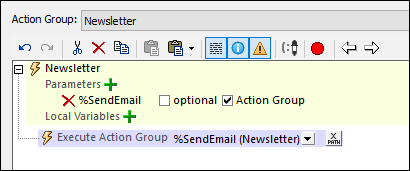You could of course combine both sets of actions.